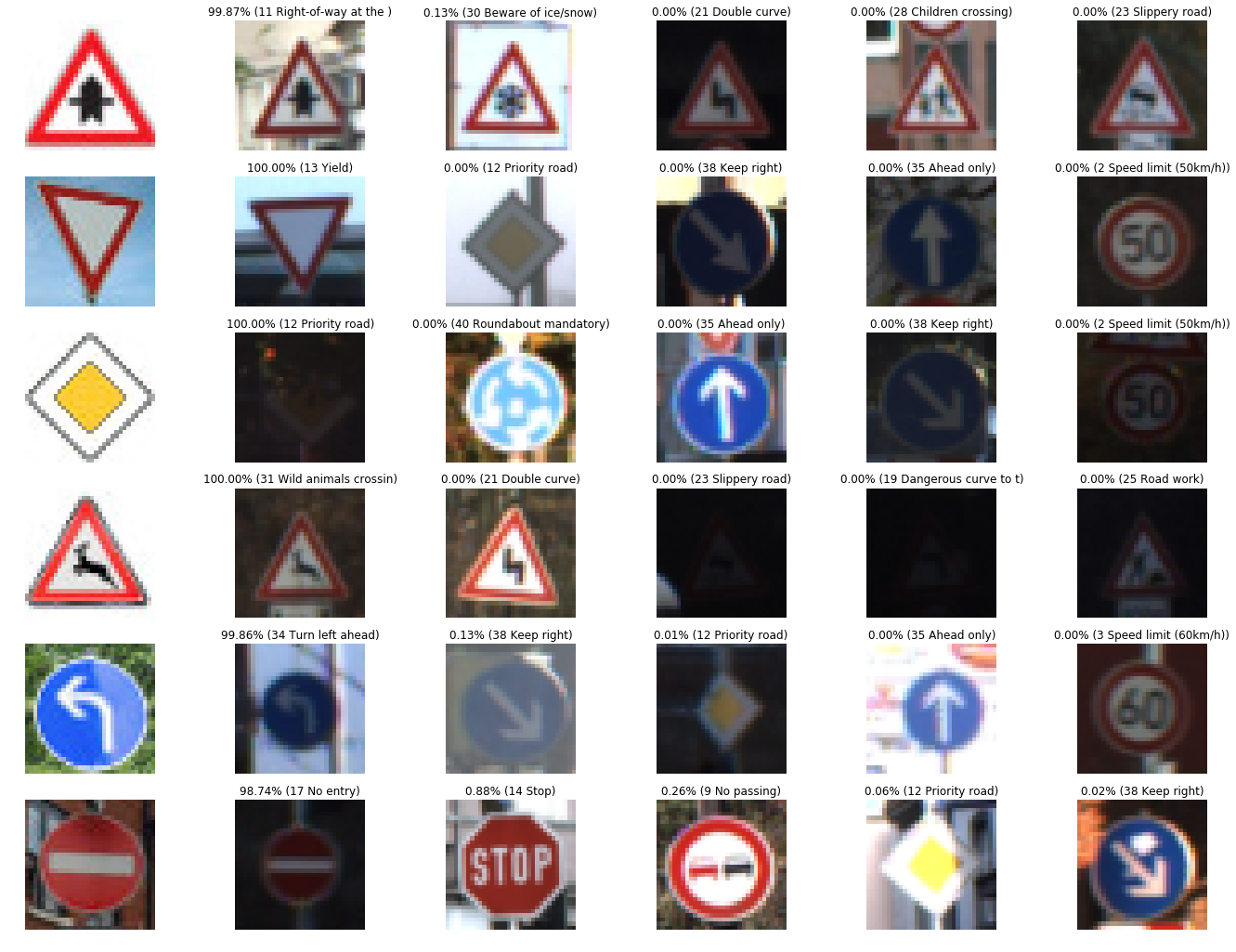# Self-Driving Car Engineer Nanodegree¶

## Project: Build a Traffic Sign Recognition Classifier¶

In this notebook, a template is provided for you to implement your functionality in stages, which is required to successfully complete this project. If additional code is required that cannot be included in the notebook, be sure that the Python code is successfully imported and included in your submission if necessary.

Note: Once you have completed all of the code implementations, you need to finalize your work by exporting the iPython Notebook as an HTML document. Before exporting the notebook to html, all of the code cells need to have been run so that reviewers can see the final implementation and output. You can then export the notebook by using the menu above and navigating to \n", "File -> Download as -> HTML (.html). Include the finished document along with this notebook as your submission.

In addition to implementing code, there is a writeup to complete. The writeup should be completed in a separate file, which can be either a markdown file or a pdf document. There is a write up template that can be used to guide the writing process. Completing the code template and writeup template will cover all of the rubric points for this project.

The rubric contains "Stand Out Suggestions" for enhancing the project beyond the minimum requirements. The stand out suggestions are optional. If you decide to pursue the "stand out suggestions", you can include the code in this Ipython notebook and also discuss the results in the writeup file.

Note: Code and Markdown cells can be executed using the Shift + Enter keyboard shortcut. In addition, Markdown cells can be edited by typically double-clicking the cell to enter edit mode.

## Step 0: Load The Data¶

In :
# Load pickled data
import pickle

traffic_signs_data_folder = 'traffic-signs-data/'
training_file = traffic_signs_data_folder + 'train.p'
validation_file= traffic_signs_data_folder + 'valid.p'
testing_file = traffic_signs_data_folder + 'test.p'

with open(training_file, mode='rb') as f:
with open(validation_file, mode='rb') as f:
with open(testing_file, mode='rb') as f:

X_train, y_train = train['features'], train['labels']
X_valid, y_valid = valid['features'], valid['labels']
X_test, y_test = test['features'], test['labels']


Data loaded


## Step 1: Dataset Summary & Exploration¶

The pickled data is a dictionary with 4 key/value pairs:

• 'features' is a 4D array containing raw pixel data of the traffic sign images, (num examples, width, height, channels).
• 'labels' is a 1D array containing the label/class id of the traffic sign. The file signnames.csv contains id -> name mappings for each id.
• 'sizes' is a list containing tuples, (width, height) representing the original width and height the image.
• 'coords' is a list containing tuples, (x1, y1, x2, y2) representing coordinates of a bounding box around the sign in the image. THESE COORDINATES ASSUME THE ORIGINAL IMAGE. THE PICKLED DATA CONTAINS RESIZED VERSIONS (32 by 32) OF THESE IMAGES

Complete the basic data summary below. Use python, numpy and/or pandas methods to calculate the data summary rather than hard coding the results. For example, the pandas shape method might be useful for calculating some of the summary results.

### Provide a Basic Summary of the Data Set Using Python, Numpy and/or Pandas¶

In :
### Replace each question mark with the appropriate value.
### Use python, pandas or numpy methods rather than hard coding the results
import pandas as pd
import numpy as np

assert (len(X_train) == len(y_train))
assert (len(X_valid) == len(y_valid))
assert (len(X_test)  == len(y_test))

# Number of training examples
n_train = len(X_train)

# Number of validation examples
n_validation = len(X_valid)

# Number of testing examples.
n_test = len(X_test)

# shape of an traffic sign image
image_shape = X_train.shape

# How many unique classes/labels there are in the dataset.
n_classes = np.unique(y_train).size

print("The size of training set is ", n_train)
print("The size of the validation set is ", n_validation)
print("The size of test set is ", n_test)
print("The shape of a traffic sign image is ", image_shape)
print("The number of unique classes/labels in the data set is =", n_classes)

The size of training set is  34799
The size of the validation set is  4410
The size of test set is  12630
The shape of a traffic sign image is  (32, 32, 3)
The number of unique classes/labels in the data set is = 43


### Include an exploratory visualization of the dataset¶

Visualize the German Traffic Signs Dataset using the pickled file(s). This is open ended, suggestions include: plotting traffic sign images, plotting the count of each sign, etc.

The Matplotlib examples and gallery pages are a great resource for doing visualizations in Python.

NOTE: It's recommended you start with something simple first. If you wish to do more, come back to it after you've completed the rest of the sections. It can be interesting to look at the distribution of classes in the training, validation and test set. Is the distribution the same? Are there more examples of some classes than others?

In :
### Data exploration visualization code goes here.
### Feel free to use as many code cells as needed.
import matplotlib.pyplot as plt
# Visualizations will be shown in the notebook.
%matplotlib inline

In :
for index in range(100,101):
image = X_train[index]
plt.figure(figsize=(1,1))
plt.imshow(image)
print(y_train[index])

41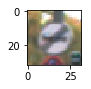In :
# read the semantics of the numbers
import csv
import random
# Visualizations will be shown in the notebook.
%matplotlib inline

classId2SignName = {}

with open('signnames.csv') as csvfile:
classId2SignName[row] = row

def visualizeImages(images,labels):
n_columns = 8
n_rows = int(len(images)/n_columns)+1
width = 24
height = n_rows * 3
fig, axs = plt.subplots(n_rows,n_columns, figsize=(width, height))
axs = axs.ravel()
for i in range(n_columns * n_rows):
axs[i].axis('off')
if (i<len(images)):
image = images[i]
axs[i].axis('off')
#if (image.shape == 1):
#    print(image)
#    axs[i].imshow(image.astype(np.uint8).squeeze, cmap='gray')
#else:
axs[i].imshow(image)
axs[i].set_title('{} ({:.20})'.format(labels[i], classId2SignName[str(labels[i])]))

print ('done')

done

In :
X = []
Y = []

labels, indices = np.unique(y_train, return_index=True)
for i in range(len(labels)):
X.append(X_train[indices[i]])
Y.append(labels[i])

visualizeImages(X,Y)

print ('done')

done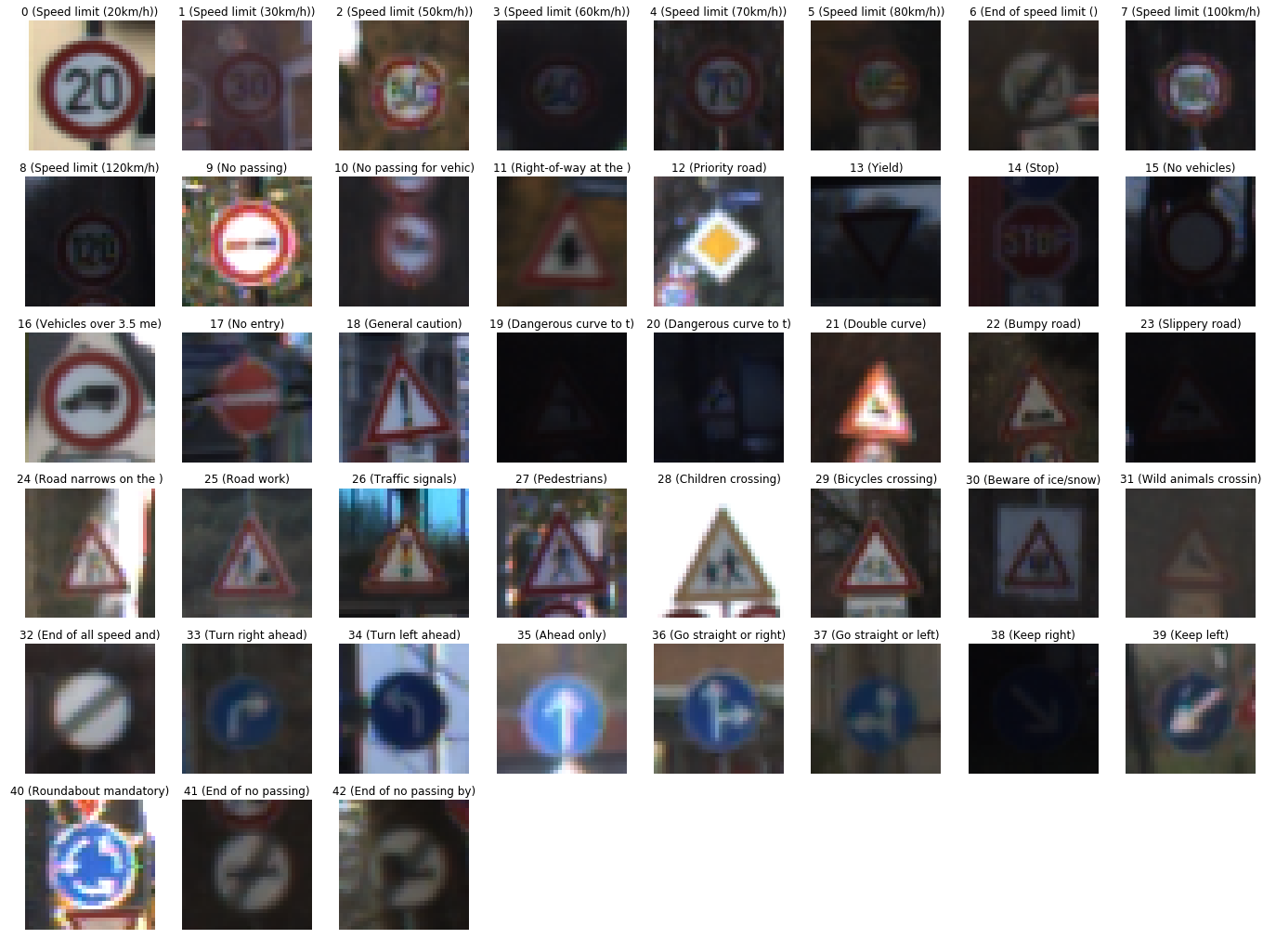In :
x,y = np.unique(y_train, return_counts=True)
plt.bar(x,y)
plt.show

print ('done')

done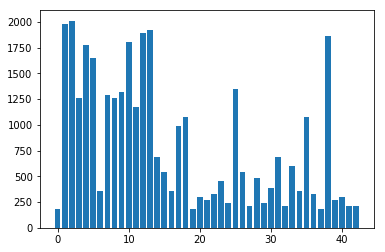## Step 2: Design and Test a Model Architecture¶

Design and implement a deep learning model that learns to recognize traffic signs. Train and test your model on the German Traffic Sign Dataset.

The LeNet-5 implementation shown in the classroom at the end of the CNN lesson is a solid starting point. You'll have to change the number of classes and possibly the preprocessing, but aside from that it's plug and play!

With the LeNet-5 solution from the lecture, you should expect a validation set accuracy of about 0.89. To meet specifications, the validation set accuracy will need to be at least 0.93. It is possible to get an even higher accuracy, but 0.93 is the minimum for a successful project submission.

• Neural network architecture (is the network over or underfitting?)
• Play around preprocessing techniques (normalization, rgb to grayscale, etc)
• Number of examples per label (some have more than others).
• Generate fake data.

Here is an example of a published baseline model on this problem. It's not required to be familiar with the approach used in the paper but, it's good practice to try to read papers like these.

### Pre-process the Data Set (normalization, grayscale, etc.)¶

Minimally, the image data should be normalized so that the data has mean zero and equal variance. For image data, (pixel - 128)/ 128 is a quick way to approximately normalize the data and can be used in this project.

Other pre-processing steps are optional. You can try different techniques to see if it improves performance.

Use the code cell (or multiple code cells, if necessary) to implement the first step of your project.

In :
### Preprocess the data here. It is required to normalize the data. Other preprocessing steps could include
### converting to grayscale, etc.
### Feel free to use as many code cells as needed.

def preProcess(color_images):
# grayscale
grayscaled_images = np.sum(color_images/3, axis=3, keepdims=True)

# normalize
normalized_images = (grayscaled_images - 128) / 128

return normalized_images

print ('done')

done

In :
def getRandomImage(x, y, filter_index):
indices, = np.nonzero( y==filter_index )
index = np.random.choice(indices)
return x[index]

image = getRandomImage(X_train, y_train, 23)
plt.figure(figsize=(1,1))
plt.imshow(image)

print ('done')

done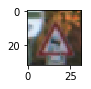In :
print (X_train.shape)
preprocessed = preProcess(X_train)
print (preprocessed.shape)

print ('done')

(34799, 32, 32, 3)
(34799, 32, 32, 1)
done


### Model Architecture¶

In :
from sklearn.utils import shuffle

X_train, y_train = shuffle(X_train, y_train)

In :
import tensorflow as tf

EPOCHS = 150
BATCH_SIZE = 128
DROPOUT = 0.5

In :
keep_prob = tf.placeholder(tf.float32)
x = tf.placeholder(tf.float32, (None, 32, 32, 1))
y = tf.placeholder(tf.int32, (None))
one_hot_y = tf.one_hot(y, 43)
keep_prob = tf.placeholder(tf.float32)

In :
from tensorflow.contrib.layers import flatten

def LeNet(x):
# Arguments used for tf.truncated_normal, randomly defines variables for the weights and biases for each layer
mu = 0
sigma = 0.1

# Layer 1: Convolutional. Input = 32x32x1. Output = 28x28x6.
conv1_W = tf.Variable(tf.truncated_normal(shape=(5, 5, 1, 6), mean = mu, stddev = sigma))
conv1_b = tf.Variable(tf.zeros(6))
conv1   = tf.nn.conv2d(x, conv1_W, strides=[1, 1, 1, 1], padding='VALID') + conv1_b

# Layer 1: Activation.
conv1 = tf.nn.relu(conv1)

# Layer 1: Pooling. Input = 28x28x6. Output = 14x14x6.
conv1 = tf.nn.max_pool(conv1, ksize=[1, 2, 2, 1], strides=[1, 2, 2, 1], padding='VALID')

# Layer 2: Convolutional. Output = 10x10x16.
conv2_W = tf.Variable(tf.truncated_normal(shape=(5, 5, 6, 16), mean = mu, stddev = sigma))
conv2_b = tf.Variable(tf.zeros(16))
conv2   = tf.nn.conv2d(conv1, conv2_W, strides=[1, 1, 1, 1], padding='VALID') + conv2_b

# Layer 2: Activation.
conv2 = tf.nn.relu(conv2)

# Layer 2: Pooling. Input = 10x10x16. Output = 5x5x16.
conv2 = tf.nn.max_pool(conv2, ksize=[1, 2, 2, 1], strides=[1, 2, 2, 1], padding='VALID')

# Layer 2: Flatten. Input = 5x5x16. Output = 400.
fc0   = flatten(conv2)

# Layer 3: Fully Connected. Input = 400. Output = 120.
fc1_W = tf.Variable(tf.truncated_normal(shape=(400, 120), mean = mu, stddev = sigma))
fc1_b = tf.Variable(tf.zeros(120))
fc1   = tf.matmul(fc0, fc1_W) + fc1_b

# Layer 3: Activation.
fc1    = tf.nn.relu(fc1)

# Layer 4: Fully Connected. Input = 120. Output = 84.
fc2_W  = tf.Variable(tf.truncated_normal(shape=(120, 84), mean = mu, stddev = sigma))
fc2_b  = tf.Variable(tf.zeros(84))
fc2    = tf.matmul(fc1, fc2_W) + fc2_b

# Layer 4: Activation.
fc2    = tf.nn.relu(fc2)

# Layer 5: Fully Connected. Input = 84. Output = 43.
fc3_W  = tf.Variable(tf.truncated_normal(shape=(84, 43), mean = mu, stddev = sigma))
fc3_b  = tf.Variable(tf.zeros(43))

logits = tf.matmul(fc2, fc3_W) + fc3_b

return logits

In :
from tensorflow.contrib.layers import flatten

def LeNet_modified(x):
# Arguments used for tf.truncated_normal, randomly defines variables for the weights and biases for each layer
mu = 0
sigma = 0.1

# Layer 1: Convolutional. Input = 32x32x1. Output = 28x28x6.
conv1_W = tf.Variable(tf.truncated_normal(shape=(5, 5, 1, 6), mean = mu, stddev = sigma))
conv1_b = tf.Variable(tf.zeros(6))
conv1   = tf.nn.conv2d(x, conv1_W, strides=[1, 1, 1, 1], padding='VALID') + conv1_b

# Layer 1: Activation.
conv1 = tf.nn.relu(conv1)

# Layer 1: Pooling. Input = 28x28x6. Output = 14x14x6.
conv1 = tf.nn.max_pool(conv1, ksize=[1, 2, 2, 1], strides=[1, 2, 2, 1], padding='VALID')

# Layer 2: Convolutional. Output = 10x10x16.
conv2_W = tf.Variable(tf.truncated_normal(shape=(5, 5, 6, 16), mean = mu, stddev = sigma))
conv2_b = tf.Variable(tf.zeros(16))
conv2   = tf.nn.conv2d(conv1, conv2_W, strides=[1, 1, 1, 1], padding='VALID') + conv2_b

# Layer 2: Activation.
conv2 = tf.nn.relu(conv2)

# Layer 2: Pooling. Input = 10x10x16. Output = 5x5x16.
conv2 = tf.nn.max_pool(conv2, ksize=[1, 2, 2, 1], strides=[1, 2, 2, 1], padding='VALID')

# Layer 2: Flatten. Input = 5x5x16. Output = 400.
fc0   = flatten(conv2)
fc0   = tf.nn.dropout(fc0, keep_prob)

# Layer 3: Fully Connected. Input = 400. Output = 120.
fc1_W = tf.Variable(tf.truncated_normal(shape=(400, 120), mean = mu, stddev = sigma))
fc1_b = tf.Variable(tf.zeros(120))
fc1   = tf.matmul(fc0, fc1_W) + fc1_b

# Layer 3: Activation.
fc1    = tf.nn.relu(fc1)
fc1    = tf.nn.dropout(fc1, keep_prob)

# Layer 4: Fully Connected. Input = 120. Output = 84.
fc2_W  = tf.Variable(tf.truncated_normal(shape=(120, 84), mean = mu, stddev = sigma))
fc2_b  = tf.Variable(tf.zeros(84))
fc2    = tf.matmul(fc1, fc2_W) + fc2_b

# Layer 4: Activation.
fc2    = tf.nn.relu(fc2)
fc2    = tf.nn.dropout(fc2, keep_prob)

# Layer 5: Fully Connected. Input = 84. Output = 43.
fc3_W  = tf.Variable(tf.truncated_normal(shape=(84, 43), mean = mu, stddev = sigma))
fc3_b  = tf.Variable(tf.zeros(43))

logits = tf.matmul(fc2, fc3_W) + fc3_b

return logits


### Train, Validate and Test the Model¶

A validation set can be used to assess how well the model is performing. A low accuracy on the training and validation sets imply underfitting. A high accuracy on the training set but low accuracy on the validation set implies overfitting.

In :
### Train your model here.
### Calculate and report the accuracy on the training and validation set.
### Once a final model architecture is selected,
### the accuracy on the test set should be calculated and reported as well.
### Feel free to use as many code cells as needed.

rate = 0.0006

logits = LeNet_modified(x)
cross_entropy = tf.nn.softmax_cross_entropy_with_logits(labels=one_hot_y, logits=logits)
loss_operation = tf.reduce_mean(cross_entropy)
training_operation = optimizer.minimize(loss_operation)

correct_prediction = tf.equal(tf.argmax(logits, 1), tf.argmax(one_hot_y, 1))
accuracy_operation = tf.reduce_mean(tf.cast(correct_prediction, tf.float32))
saver = tf.train.Saver()

def evaluate(X_data, y_data):
num_examples = len(X_data)
total_accuracy = 0
sess = tf.get_default_session()
for offset in range(0, num_examples, BATCH_SIZE):
batch_x, batch_y = X_data[offset:offset+BATCH_SIZE], y_data[offset:offset+BATCH_SIZE]
accuracy = sess.run(accuracy_operation, feed_dict={x: batch_x, y: batch_y, keep_prob: 1.0})
total_accuracy += (accuracy * len(batch_x))

In :
X_train_preProcessed = preProcess(X_train)
X_valid_preProcessed = preProcess(X_valid)
X_test_preProcessed  = preProcess(X_test)

print ('done')

done

In :
with tf.Session() as sess:
sess.run(tf.global_variables_initializer())
num_examples = len(X_train)

print("Training...")
print()
validation_accuracies = []

for i in range(EPOCHS):
X_train, X_train_preProcessed, y_train = shuffle(X_train, X_train_preProcessed, y_train)
for offset in range(0, num_examples, BATCH_SIZE):
end = offset + BATCH_SIZE
batch_x, batch_y = X_train_preProcessed[offset:end], y_train[offset:end]
sess.run(training_operation, feed_dict={x: batch_x, y: batch_y, keep_prob: DROPOUT})

training_accuracy = evaluate(X_train_preProcessed, y_train)
validation_accuracy = evaluate(X_valid_preProcessed, y_valid)

print("EPOCH {} ...".format(i+1))
print("Training Accuracy = {:.3f}".format(training_accuracy))
print("Validation Accuracy = {:.3f}".format(validation_accuracy))
print()
validation_accuracies.append(validation_accuracy)

saver.save(sess, './lenet')

# show learning statistics
plt.plot(range(EPOCHS),validation_accuracies)
plt.show

print("Model saved")

Training...

EPOCH 1 ...
Training Accuracy = 0.322
Validation Accuracy = 0.268

EPOCH 2 ...
Training Accuracy = 0.566
Validation Accuracy = 0.519

EPOCH 3 ...
Training Accuracy = 0.703
Validation Accuracy = 0.657

EPOCH 4 ...
Training Accuracy = 0.783
Validation Accuracy = 0.737

EPOCH 5 ...
Training Accuracy = 0.825
Validation Accuracy = 0.768

EPOCH 6 ...
Training Accuracy = 0.868
Validation Accuracy = 0.823

EPOCH 7 ...
Training Accuracy = 0.876
Validation Accuracy = 0.836

EPOCH 8 ...
Training Accuracy = 0.901
Validation Accuracy = 0.854

EPOCH 9 ...
Training Accuracy = 0.904
Validation Accuracy = 0.856

EPOCH 10 ...
Training Accuracy = 0.917
Validation Accuracy = 0.874

EPOCH 11 ...
Training Accuracy = 0.924
Validation Accuracy = 0.879

EPOCH 12 ...
Training Accuracy = 0.925
Validation Accuracy = 0.890

EPOCH 13 ...
Training Accuracy = 0.932
Validation Accuracy = 0.896

EPOCH 14 ...
Training Accuracy = 0.936
Validation Accuracy = 0.897

EPOCH 15 ...
Training Accuracy = 0.944
Validation Accuracy = 0.895

EPOCH 16 ...
Training Accuracy = 0.945
Validation Accuracy = 0.900

EPOCH 17 ...
Training Accuracy = 0.945
Validation Accuracy = 0.899

EPOCH 18 ...
Training Accuracy = 0.951
Validation Accuracy = 0.908

EPOCH 19 ...
Training Accuracy = 0.955
Validation Accuracy = 0.908

EPOCH 20 ...
Training Accuracy = 0.954
Validation Accuracy = 0.902

EPOCH 21 ...
Training Accuracy = 0.961
Validation Accuracy = 0.917

EPOCH 22 ...
Training Accuracy = 0.959
Validation Accuracy = 0.921

EPOCH 23 ...
Training Accuracy = 0.963
Validation Accuracy = 0.918

EPOCH 24 ...
Training Accuracy = 0.968
Validation Accuracy = 0.925

EPOCH 25 ...
Training Accuracy = 0.963
Validation Accuracy = 0.919

EPOCH 26 ...
Training Accuracy = 0.972
Validation Accuracy = 0.929

EPOCH 27 ...
Training Accuracy = 0.971
Validation Accuracy = 0.932

EPOCH 28 ...
Training Accuracy = 0.974
Validation Accuracy = 0.933

EPOCH 29 ...
Training Accuracy = 0.975
Validation Accuracy = 0.934

EPOCH 30 ...
Training Accuracy = 0.972
Validation Accuracy = 0.930

EPOCH 31 ...
Training Accuracy = 0.978
Validation Accuracy = 0.939

EPOCH 32 ...
Training Accuracy = 0.979
Validation Accuracy = 0.935

EPOCH 33 ...
Training Accuracy = 0.980
Validation Accuracy = 0.940

EPOCH 34 ...
Training Accuracy = 0.980
Validation Accuracy = 0.939

EPOCH 35 ...
Training Accuracy = 0.979
Validation Accuracy = 0.940

EPOCH 36 ...
Training Accuracy = 0.981
Validation Accuracy = 0.945

EPOCH 37 ...
Training Accuracy = 0.982
Validation Accuracy = 0.943

EPOCH 38 ...
Training Accuracy = 0.982
Validation Accuracy = 0.944

EPOCH 39 ...
Training Accuracy = 0.985
Validation Accuracy = 0.944

EPOCH 40 ...
Training Accuracy = 0.985
Validation Accuracy = 0.949

EPOCH 41 ...
Training Accuracy = 0.985
Validation Accuracy = 0.950

EPOCH 42 ...
Training Accuracy = 0.986
Validation Accuracy = 0.951

EPOCH 43 ...
Training Accuracy = 0.982
Validation Accuracy = 0.944

EPOCH 44 ...
Training Accuracy = 0.987
Validation Accuracy = 0.951

EPOCH 45 ...
Training Accuracy = 0.986
Validation Accuracy = 0.953

EPOCH 46 ...
Training Accuracy = 0.988
Validation Accuracy = 0.952

EPOCH 47 ...
Training Accuracy = 0.988
Validation Accuracy = 0.954

EPOCH 48 ...
Training Accuracy = 0.987
Validation Accuracy = 0.951

EPOCH 49 ...
Training Accuracy = 0.988
Validation Accuracy = 0.947

EPOCH 50 ...
Training Accuracy = 0.990
Validation Accuracy = 0.953

EPOCH 51 ...
Training Accuracy = 0.988
Validation Accuracy = 0.951

EPOCH 52 ...
Training Accuracy = 0.988
Validation Accuracy = 0.955

EPOCH 53 ...
Training Accuracy = 0.990
Validation Accuracy = 0.956

EPOCH 54 ...
Training Accuracy = 0.990
Validation Accuracy = 0.954

EPOCH 55 ...
Training Accuracy = 0.982
Validation Accuracy = 0.941

EPOCH 56 ...
Training Accuracy = 0.990
Validation Accuracy = 0.957

EPOCH 57 ...
Training Accuracy = 0.991
Validation Accuracy = 0.958

EPOCH 58 ...
Training Accuracy = 0.991
Validation Accuracy = 0.956

EPOCH 59 ...
Training Accuracy = 0.990
Validation Accuracy = 0.954

EPOCH 60 ...
Training Accuracy = 0.991
Validation Accuracy = 0.955

EPOCH 61 ...
Training Accuracy = 0.991
Validation Accuracy = 0.956

EPOCH 62 ...
Training Accuracy = 0.991
Validation Accuracy = 0.958

EPOCH 63 ...
Training Accuracy = 0.992
Validation Accuracy = 0.959

EPOCH 64 ...
Training Accuracy = 0.991
Validation Accuracy = 0.959

EPOCH 65 ...
Training Accuracy = 0.992
Validation Accuracy = 0.960

EPOCH 66 ...
Training Accuracy = 0.992
Validation Accuracy = 0.959

EPOCH 67 ...
Training Accuracy = 0.992
Validation Accuracy = 0.967

EPOCH 68 ...
Training Accuracy = 0.992
Validation Accuracy = 0.966

EPOCH 69 ...
Training Accuracy = 0.992
Validation Accuracy = 0.961

EPOCH 70 ...
Training Accuracy = 0.991
Validation Accuracy = 0.962

EPOCH 71 ...
Training Accuracy = 0.992
Validation Accuracy = 0.963

EPOCH 72 ...
Training Accuracy = 0.993
Validation Accuracy = 0.960

EPOCH 73 ...
Training Accuracy = 0.993
Validation Accuracy = 0.963

EPOCH 74 ...
Training Accuracy = 0.993
Validation Accuracy = 0.963

EPOCH 75 ...
Training Accuracy = 0.993
Validation Accuracy = 0.958

EPOCH 76 ...
Training Accuracy = 0.993
Validation Accuracy = 0.963

EPOCH 77 ...
Training Accuracy = 0.993
Validation Accuracy = 0.960

EPOCH 78 ...
Training Accuracy = 0.993
Validation Accuracy = 0.961

EPOCH 79 ...
Training Accuracy = 0.994
Validation Accuracy = 0.965

EPOCH 80 ...
Training Accuracy = 0.993
Validation Accuracy = 0.961

EPOCH 81 ...
Training Accuracy = 0.993
Validation Accuracy = 0.961

EPOCH 82 ...
Training Accuracy = 0.993
Validation Accuracy = 0.969

EPOCH 83 ...
Training Accuracy = 0.993
Validation Accuracy = 0.967

EPOCH 84 ...
Training Accuracy = 0.994
Validation Accuracy = 0.967

EPOCH 85 ...
Training Accuracy = 0.993
Validation Accuracy = 0.960

EPOCH 86 ...
Training Accuracy = 0.994
Validation Accuracy = 0.964

EPOCH 87 ...
Training Accuracy = 0.994
Validation Accuracy = 0.964

EPOCH 88 ...
Training Accuracy = 0.993
Validation Accuracy = 0.961

EPOCH 89 ...
Training Accuracy = 0.994
Validation Accuracy = 0.968

EPOCH 90 ...
Training Accuracy = 0.994
Validation Accuracy = 0.964

EPOCH 91 ...
Training Accuracy = 0.995
Validation Accuracy = 0.965

EPOCH 92 ...
Training Accuracy = 0.995
Validation Accuracy = 0.968

EPOCH 93 ...
Training Accuracy = 0.993
Validation Accuracy = 0.963

EPOCH 94 ...
Training Accuracy = 0.994
Validation Accuracy = 0.965

EPOCH 95 ...
Training Accuracy = 0.992
Validation Accuracy = 0.969

EPOCH 96 ...
Training Accuracy = 0.994
Validation Accuracy = 0.971

EPOCH 97 ...
Training Accuracy = 0.995
Validation Accuracy = 0.969

EPOCH 98 ...
Training Accuracy = 0.994
Validation Accuracy = 0.966

EPOCH 99 ...
Training Accuracy = 0.994
Validation Accuracy = 0.968

EPOCH 100 ...
Training Accuracy = 0.994
Validation Accuracy = 0.965

EPOCH 101 ...
Training Accuracy = 0.995
Validation Accuracy = 0.967

EPOCH 102 ...
Training Accuracy = 0.995
Validation Accuracy = 0.971

EPOCH 103 ...
Training Accuracy = 0.993
Validation Accuracy = 0.971

EPOCH 104 ...
Training Accuracy = 0.995
Validation Accuracy = 0.967

EPOCH 105 ...
Training Accuracy = 0.992
Validation Accuracy = 0.959

EPOCH 106 ...
Training Accuracy = 0.995
Validation Accuracy = 0.965

EPOCH 107 ...
Training Accuracy = 0.994
Validation Accuracy = 0.970

EPOCH 108 ...
Training Accuracy = 0.995
Validation Accuracy = 0.968

EPOCH 109 ...
Training Accuracy = 0.994
Validation Accuracy = 0.965

EPOCH 110 ...
Training Accuracy = 0.995
Validation Accuracy = 0.968

EPOCH 111 ...
Training Accuracy = 0.994
Validation Accuracy = 0.966

EPOCH 112 ...
Training Accuracy = 0.995
Validation Accuracy = 0.971

EPOCH 113 ...
Training Accuracy = 0.995
Validation Accuracy = 0.971

EPOCH 114 ...
Training Accuracy = 0.994
Validation Accuracy = 0.968

EPOCH 115 ...
Training Accuracy = 0.996
Validation Accuracy = 0.972

EPOCH 116 ...
Training Accuracy = 0.995
Validation Accuracy = 0.970

EPOCH 117 ...
Training Accuracy = 0.996
Validation Accuracy = 0.968

EPOCH 118 ...
Training Accuracy = 0.995
Validation Accuracy = 0.968

EPOCH 119 ...
Training Accuracy = 0.994
Validation Accuracy = 0.966

EPOCH 120 ...
Training Accuracy = 0.996
Validation Accuracy = 0.968

EPOCH 121 ...
Training Accuracy = 0.995
Validation Accuracy = 0.974

EPOCH 122 ...
Training Accuracy = 0.995
Validation Accuracy = 0.968

EPOCH 123 ...
Training Accuracy = 0.995
Validation Accuracy = 0.972

EPOCH 124 ...
Training Accuracy = 0.996
Validation Accuracy = 0.969

EPOCH 125 ...
Training Accuracy = 0.995
Validation Accuracy = 0.975

EPOCH 126 ...
Training Accuracy = 0.995
Validation Accuracy = 0.970

EPOCH 127 ...
Training Accuracy = 0.995
Validation Accuracy = 0.972

EPOCH 128 ...
Training Accuracy = 0.995
Validation Accuracy = 0.973

EPOCH 129 ...
Training Accuracy = 0.995
Validation Accuracy = 0.974

EPOCH 130 ...
Training Accuracy = 0.996
Validation Accuracy = 0.972

EPOCH 131 ...
Training Accuracy = 0.994
Validation Accuracy = 0.972

EPOCH 132 ...
Training Accuracy = 0.996
Validation Accuracy = 0.973

EPOCH 133 ...
Training Accuracy = 0.995
Validation Accuracy = 0.971

EPOCH 134 ...
Training Accuracy = 0.995
Validation Accuracy = 0.974

EPOCH 135 ...
Training Accuracy = 0.995
Validation Accuracy = 0.971

EPOCH 136 ...
Training Accuracy = 0.993
Validation Accuracy = 0.969

EPOCH 137 ...
Training Accuracy = 0.995
Validation Accuracy = 0.971

EPOCH 138 ...
Training Accuracy = 0.996
Validation Accuracy = 0.974

EPOCH 139 ...
Training Accuracy = 0.996
Validation Accuracy = 0.973

EPOCH 140 ...
Training Accuracy = 0.996
Validation Accuracy = 0.975

EPOCH 141 ...
Training Accuracy = 0.996
Validation Accuracy = 0.973

EPOCH 142 ...
Training Accuracy = 0.996
Validation Accuracy = 0.970

EPOCH 143 ...
Training Accuracy = 0.993
Validation Accuracy = 0.964

EPOCH 144 ...
Training Accuracy = 0.996
Validation Accuracy = 0.972

EPOCH 145 ...
Training Accuracy = 0.996
Validation Accuracy = 0.973

EPOCH 146 ...
Training Accuracy = 0.996
Validation Accuracy = 0.972

EPOCH 147 ...
Training Accuracy = 0.995
Validation Accuracy = 0.974

EPOCH 148 ...
Training Accuracy = 0.997
Validation Accuracy = 0.972

EPOCH 149 ...
Training Accuracy = 0.996
Validation Accuracy = 0.969

EPOCH 150 ...
Training Accuracy = 0.996
Validation Accuracy = 0.975

Model saved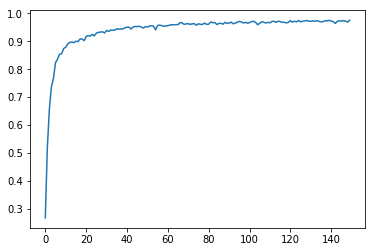In :
with tf.Session() as sess:
saver.restore(sess, tf.train.latest_checkpoint('.'))

test_accuracy = evaluate(X_test_preProcessed, y_test)
print("Test Accuracy = {:.3f}".format(test_accuracy))

Test Accuracy = 0.951


## Step 3: Test a Model on New Images¶

To give yourself more insight into how your model is working, download at least five pictures of German traffic signs from the web and use your model to predict the traffic sign type.

You may find signnames.csv useful as it contains mappings from the class id (integer) to the actual sign name.

### Load and Output the Images¶

In :
### Load the images and plot them here.
### Feel free to use as many code cells as needed.
import numpy as np
import matplotlib.pyplot as plt
import matplotlib.image as mpimg
import cv2
import glob

traffic_signs_data_folder = 'test_data/'

my_X_test = []
my_Y_test = []
for image_file in glob.glob(traffic_signs_data_folder + "*.jpg"):
try:
#if (True):
# the label is encoded into the first two characters of the file names
label_str = image_file[len(traffic_signs_data_folder):][:2]
label = int(label_str)
my_X_test.append(image)
my_Y_test.append(label)
except  Exception:
print ('Ignoring image {}'.format(image_file))

visualizeImages(my_X_test, my_Y_test)

print ('Done')

Done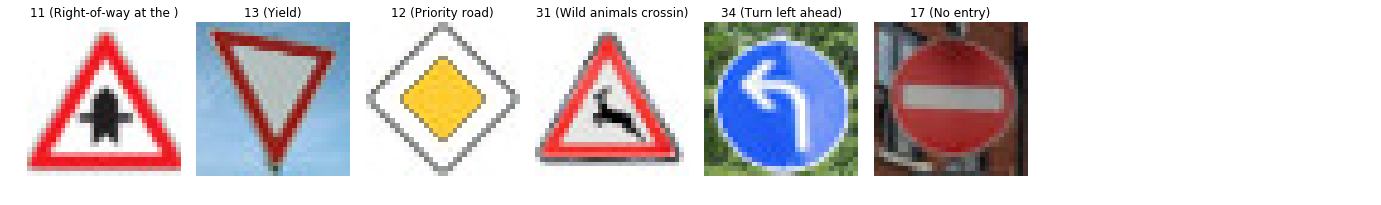### Predict the Sign Type for Each Image¶

In :
### Run the predictions here and use the model to output the prediction for each image.
### Make sure to pre-process the images with the same pre-processing pipeline used earlier.
### Feel free to use as many code cells as needed.

my_X_test_preProcessed = preProcess(np.array(my_X_test))

prediction=tf.argmax(logits,1)

with tf.Session() as sess:
saver.restore(sess, tf.train.latest_checkpoint('.'))
predictions = sess.run(prediction, feed_dict={x: my_X_test_preProcessed, keep_prob: 1.0})
visualizeImages(my_X_test, predictions)

print (predictions)

[11 13 12 31 34 17]### Analyze Performance¶

In :
### Calculate the accuracy for these 5 new images.
### For example, if the model predicted 1 out of 5 signs correctly, it's 20% accurate on these new images.

with tf.Session() as sess:
saver.restore(sess, tf.train.latest_checkpoint('.'))

test_accuracy = evaluate(my_X_test_preProcessed, my_Y_test)
print("Test Accuracy = {:.3f}".format(test_accuracy))

Test Accuracy = 1.000


### Output Top 5 Softmax Probabilities For Each Image Found on the Web¶

For each of the new images, print out the model's softmax probabilities to show the certainty of the model's predictions (limit the output to the top 5 probabilities for each image). tf.nn.top_k could prove helpful here.

The example below demonstrates how tf.nn.top_k can be used to find the top k predictions for each image.

tf.nn.top_k will return the values and indices (class ids) of the top k predictions. So if k=3, for each sign, it'll return the 3 largest probabilities (out of a possible 43) and the correspoding class ids.

Take this numpy array as an example. The values in the array represent predictions. The array contains softmax probabilities for five candidate images with six possible classes. tf.nn.top_k is used to choose the three classes with the highest probability:

# (5, 6) array
a = np.array([[ 0.24879643,  0.07032244,  0.12641572,  0.34763842,  0.07893497,
0.12789202],
[ 0.28086119,  0.27569815,  0.08594638,  0.0178669 ,  0.18063401,
0.15899337],
[ 0.26076848,  0.23664738,  0.08020603,  0.07001922,  0.1134371 ,
0.23892179],
[ 0.11943333,  0.29198961,  0.02605103,  0.26234032,  0.1351348 ,
0.16505091],
[ 0.09561176,  0.34396535,  0.0643941 ,  0.16240774,  0.24206137,
0.09155967]])

Running it through sess.run(tf.nn.top_k(tf.constant(a), k=3)) produces:

TopKV2(values=array([[ 0.34763842,  0.24879643,  0.12789202],
[ 0.28086119,  0.27569815,  0.18063401],
[ 0.26076848,  0.23892179,  0.23664738],
[ 0.29198961,  0.26234032,  0.16505091],
[ 0.34396535,  0.24206137,  0.16240774]]), indices=array([[3, 0, 5],
[0, 1, 4],
[0, 5, 1],
[1, 3, 5],
[1, 4, 3]], dtype=int32))

Looking just at the first row we get [ 0.34763842, 0.24879643, 0.12789202], you can confirm these are the 3 largest probabilities in a. You'll also notice [3, 0, 5] are the corresponding indices.

In :
def visualizeTop_k(test_images, train_images, top_k):
probabilities = top_k.values
indices = top_k.indices
assert (len(test_images) == len(indices))

n_columns = 6
n_rows = len(indices)
width = 24
height = n_rows * 3
fig, axs = plt.subplots(n_rows,n_columns, figsize=(width, height))
axs = axs.ravel()
i = 0
for row in range(n_rows):
axs[i].axis('off')
axs[i].imshow(test_images[row])
#axs[i].set_title('{} ({:.20})'.format(labels[i], classId2SignName[str(labels[i])]))
i += 1
for j in range(len(indices[row])):
index = indices[row][j]
probability = probabilities[row][j]
axs[i].axis('off')
axs[i].set_title('{:.2f}% ({} {:.20})'.format(probability*100, index,  classId2SignName[str(index)]))
axs[i].imshow(getRandomImage(X_train, y_train, index))
i += 1

print('done')

done

In :
def visualizeTop_k2(test_images, train_images, top_k):
probabilities = top_k.values
indices = top_k.indices
assert (len(test_images) == len(indices))

n_columns = 2
n_rows = len(indices)
width = 24
height = n_rows * 3
fig, axs = plt.subplots(n_rows,n_columns, figsize=(width, height))
axs = axs.ravel()
i = 0
for row in range(n_rows):
axs[i].axis('off')
axs[i].imshow(test_images[row])
i += 1
labs=[classId2SignName[str(j)] for j in indices[row]]
axs[i].barh(labs, probabilities[row])

#axs[i].yticks(np.arange(1, 6, 1), labs)
i += 1

print('done')

done

In :
### Print out the top five softmax probabilities for the predictions on the German traffic sign images found on the web.
### Feel free to use as many code cells as needed.
softmax_logits = tf.nn.softmax(logits)
top_k=tf.nn.top_k(softmax_logits,5)

with tf.Session() as sess:
saver.restore(sess, tf.train.latest_checkpoint('.'))
top_k = sess.run(top_k, feed_dict={x: my_X_test_preProcessed, keep_prob : 1.0})

visualizeTop_k(my_X_test, X_train, top_k)
visualizeTop_k2(my_X_test, X_train, top_k)

print('done')

done